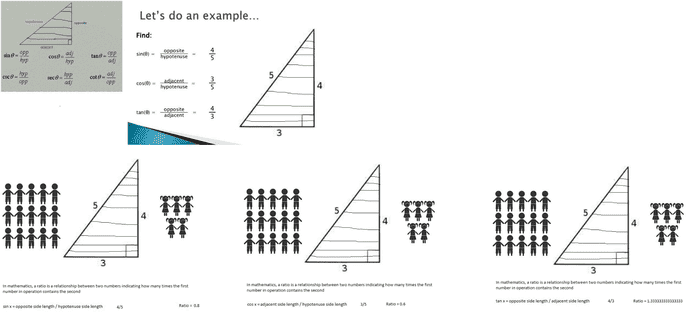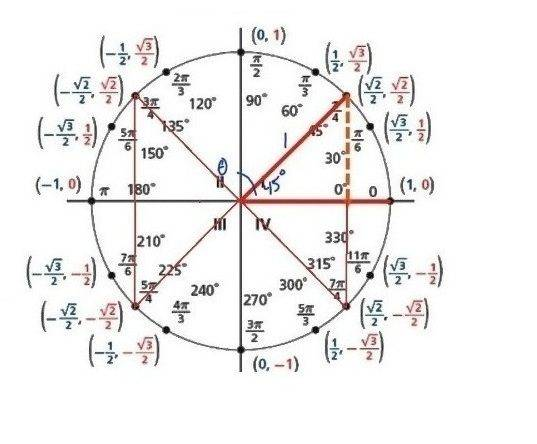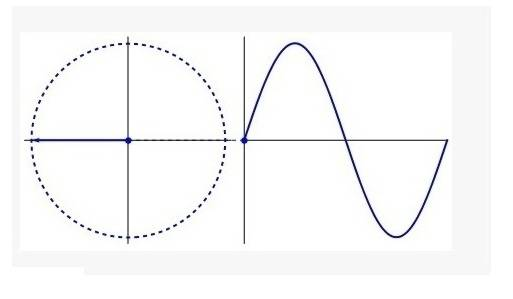# In trigonometry, what is the period of a wave?

what does the period of a wave , in trigonometry ... depend on ?

does it depend on the ratio of the length of the sides of the right angled triangle like this ?does it depend on the angle ??what are all the values you need to plot a graph like this ???

Last edited:

ProfuselyQuarky
Gold Member
Sinusoidal functions are often in the format ##y=a \sin {(bx+c)}+d## and ##y=a \cos {(bx+c)}+d##. The period of these functions (a complete cycle of part of the function) is ##\frac {2\pi}{|b|}##.

Last edited:
thanks a lot for explaining few advanced things...

so ,

i should learn functions like these ...

f(x) = a sin ( bx + c ) + d
or
y = a sin ( bx + c ) + d

and

f(x) = a cos ( bx + c ) + d
or
y = a cos ( bx + c ) + d

what does those each alphabet , means in those equations ?

any good tutorials or books ??

ProfuselyQuarky
Gold Member
It's not really "advanced" in the sense that it's overly complicated to learn. Sinusoidal functions are simply functions (generally sine and cosine functions) that have the ability to be stretched, compressed, shifted, etc. For the functions ##y=a \sin {(bx+c)}+d## and ##y=a \cos {(bx+c)}+d##:

Amplitude is ##|a|##
Period is ##\frac {2\pi}{|b|}##
Frequency is ##\frac {|b|}{2\pi}##
Phase shift is ##\frac {-c}{|b|}##
Vertical shirt is ##d##

I'm afraid that I don't know many books, though.

thanks ,

there is lot to learn ...

ProfuselyQuarky
Gold Member
thanks ,

there is lot to learn ...
There's always a lot to learn, but if we ran out of things to learn, life would be pretty boring so be happy about thatBy the way (this is often the tendency in electrical engineering courses, particularly in the time domain), you have much less to memorize if you always write any sinusoid as ##f(t) = A \sin(2\pi f t + \phi)## or ##g(t) = A\cos(2\pi f t + \phi)##. What's the period? Simply one over the frequency: ##T = 1/f##.
Example: ##f(t) = \sin(5t)##. What's the period? Simply try to get it in the form given above, and rewrite as ##f(t) = \sin(2 \pi \frac{5}{2 \pi} t)##, then we can clearly see ##f=\frac{5}{2 \pi}##, or ##T = \frac{2 \pi}{5}##. Even though it's the same thing, I personally have found it easier to simply remember the form of the sinusoid instead of a bunch of formulas.

By the way, @ProfuselyQuarky , there's a bit of a subtle nuance here. Typically, when a scientist or engineer refers to the phase shift of a sinusoid, say, of the form ##y(t) = \sin(2 \pi f t + \phi)##, they're specifically referring to ##\phi##, i.e. some constant shift to the phase. Another way of thinking about it is that at ##t = 0## (or ##x = 0## or whatever), the sinusoid is shifted from where it should be. Notice that the units would actually be different with your formula--we would be measuring the phase shift in seconds with regards to my sinusoid, whereas ##\phi## alone is in radians. It's not that it doesn't make sense to do that; it's just that we're typically interested in the former definition of phase shift, in my experience (I've never had to use your formula).

Here's an example. Suppose you send a sinusoidal wave to a friend, for fun. Assume you broadcast it with an antenna. On the way, it reflects off a building and a second wave arrives to your friend ##T## seconds later than the first one. So the total wave you friend receives is $$s(t) = \sin(2 \pi f t) + \sin(2 \pi f (t + T)) = \sin(2 \pi f t) + \sin(2 \pi f t + 2 \pi f T)$$ Here, we say ##\phi = 2 \pi f T##. The second wave is phase-shifted so that its peaks, valleys, etc. are slightly behind that of the first. Using your definition, we see that the phase shift is ##\Delta \phi = \frac{2 \pi f T}{2 \pi f} = T##. So that's just the time difference between the arrival of the waves. So you're not wrong--the definitions are basically equivalent. In my experience with coursework problems, at least, though, saying the phase shift is ##\phi = 2 \pi f T## is generally more useful.

Last edited:
•ProfuselyQuarky
ProfuselyQuarky
Gold Member
@axmls Thanks for the insight. I don't know anything about engineering worth beans so that's good to know. The formula I know is simply the one I learned when taking trig :P

@axmls Thanks for the insight. I don't know anything about engineering worth beans so that's good to know. The formula I know is simply the one I learned when taking trig :P

Well it's not exclusive to engineering. It was just a real life example. You'll find as you continue in your studies, especially if you study physics or engineering, that the phase shift texts are referring to is just that constant added to the time-dependent (or space-dependent) part of the sinusoid.

•ProfuselyQuarky
There's always a lot to learn, but if we ran out of things to learn, life would be pretty boring so be happy about thatthanks a lot for the answers ... i was kind of getting stuck ...
many of the things you and axmls talked , looked a bit too advanced for me ...

but was interesting too at the same time ...

so i have few more questions to ask about the wave ...

It's not really "advanced" in the sense that it's overly complicated to learn. Sinusoidal functions are simply functions (generally sine and cosine functions) that have the ability to be stretched, compressed, shifted, etc. For the functions ##y=a \sin {(bx+c)}+d## and ##y=a \cos {(bx+c)}+d##:

Amplitude is ##|a|##
Period is ##\frac {2\pi}{|b|}##
Frequency is ##\frac {|b|}{2\pi}##
Phase shift is ##\frac {-c}{|b|}##
Vertical shift is ##d##

I'm afraid that I don't know many books, though.

that's like a lot of information about a wave ??

could somebody answer a few basic questions for me ...?

what is that whole cycle between a crest and a trough called ??

https://i.imgsafe.org/1ae8c045eb.jpg [Broken]

what influences .. the outcome of the wave like pattern in a graph ??

https://i.imgsafe.org/1c1f9582f6.png [Broken]

??

Last edited by a moderator:
ProfuselyQuarky
Gold Member
The distance from crest to crest and trough to trough is one wave period (##\frac {2\pi}{|b|}##). The distance from crest to trough or trough to crest of 1/2 of the period .
what influences .. the outcome of the wave like pattern in a graph ??
I already said that the functions are generally in the format ##y=a\sin{(bx+c)}+d## and ##y=a\sin{(bx+c)}+d##. It is the values of those variables that determines the way the graphs look.

Last edited:
thanks again ...

then comes the period itself ...

The Period is the length from one peak to the next (or from any point to the next matching point):

the amplitude ...

The Amplitude is the height from the center line to the peak (or to the trough)

Frequency

Frequency is how often something happens per unit of time (per "1")

Phase shift

The Phase Shift is how far the function is horizontally to the right of the usual position

Vertical shift

The Vertical Shift is how far the function is vertically from the usual position

https://i.imgsafe.org/29754d6410.png [Broken]

??

i am not sure , where to from here ...

Last edited by a moderator:
ProfuselyQuarky
Gold Member
Are you ultimately trying to figure out how the right triangle relates to the unit circle and sine/cosine graphs? Is that it? Like on the images you posted above, you can see how right triangles can be found in a unit circle. You know how when you graph something each point is represented as ##(x,y)##? On a unit circle, we can see those same points as ##(\cos{t},\sin{t})##. To derive, say, a sine graph, we can take a Cartesian plane (a basic graphing plane) and replace the y-axis with ##\sin{\theta}## and the x-axis with ##\theta##. Now all we have to do is plot the points.

So, sticking to our sine graph (but cosine graphs are done the same way), when ##\theta=0^\circ## or radians, what is the value of sine? Remember how on a unit circle ##(x,y)## is the same as ##(\cos{t},\sin{t})##? So when ##\theta=0^\circ## on a unit circle, the coordinates are ##(1,0)## implying that ##\sin{0}## is 0, also. Thus, we have the first coordinate of out sine wave to be ##(0,0)##. Congratulations! We can continue to do this.....when ##\theta=30^\circ##, or ##\frac{\pi}{6}## in radians, the point on the unit circle is ##(\frac{\sqrt{3}}{2},\frac{1}{2})##, telling us that ##\sin{30^\circ}=\frac{1}{2}##. Now we've got our second point for our sine graph: ##(30,0.5)##. If we keep doing this for the entire circle, we will eventually get one period of the sine wave. Hopefully this guy can illustrate it a bit more:

Last edited:
Are you ultimately trying to figure out how the right triangle relates to the unit circle and sine/cosine graphs? Is that it? Like on the images you posted above, you can see how right triangles can be found in a unit circle. You know how when you graph something each point is represented as ##(x,y)##? On a unit circle, we can see those same points as ##(\cos{t},\sin{t})##. To derive, say, a sine graph, we can take a Cartesian plane (a basic graphing plane) and replace the y-axis with ##\sin{\theta}## and the x-axis with ##\theta##. Now all we have to do is plot the points.

So, sticking to our sine graph (but cosine graphs are done the same way), when ##\theta=0^\circ## or radians, what is the value of sine? Remember how on a unit circle ##(x,y)## is the same as ##(\cos{t},\sin{t})##? So when ##\theta=0^\circ## on a unit circle, the coordinates are ##(1,0)## implying that ##\sin{0}## is 0, also. Thus, we have the first coordinate of out sine wave to be ##(0,0)##. Congratulations! We can continue to do this.....when ##\theta=30^\circ##, or ##\frac{\pi}{6}## in radians, the point on the unit circle is ##(\frac{\sqrt{3}}{2},\frac{1}{2})##, telling us that ##\sin{30^\circ}=\frac{1}{2}##. Now we've got our second point for our sine graph: ##(30,0.5)##. If we keep doing this for the entire circle, we will eventually get one period of the sine wave. Hopefully this guy can illustrate it a bit more:

ProfuselyQuarky ,

i am really trying to see a bigger picture of trigonometry ... i had a very hard time trying to understand it from the basics , to seeing a few advanced trigonometric functions ...

which is why i keep putting pictures like these ... so that in the end , it stays in my mind ... properly ... like a big whole picture of the things i am trying to do ...

anyway , i have managed to make some proper progress in terms of understanding trigonometry ... from the basics to some advanced things ...

now , i am a little bit happy about it too ... i sort of see where this is all going ....

https://i.imgsafe.org/516f7b1670.jpg [Broken]

that is not all , i am a computer science student ... and in the end i had to write programs involving trigonometric functions and numerical methods associated with it ... in a nice compiler environment like this ...

from some basic trigonometry questions ... to a few advanced like this ...

f(x) = a sin ( bx + c ) + d
or
y = a sin ( bx + c ) + d

and

f(x) = a cos ( bx + c ) + d
or
y = a cos ( bx + c ) + d

https://i.imgsafe.org/53fa183370.png [Broken]

thanks a lot for the video ... i will try to keep on improving ...
if i have more doubts , i will start a separate thread ...
this discussion was very helpful ...

Last edited by a moderator:
ProfuselyQuarky
Gold Member
No problemMark44
Mentor
ProfuselyQuarky ,

i am really trying to see a bigger picture of trigonometry ... i had a very hard time trying to understand it from the basics , to seeing a few advanced trigonometric functions ...

which is why i keep putting pictures like these ... so that in the end , it stays in my mind ... properly ... like a big whole picture of the things i am trying to do ...

anyway , i have managed to make some proper progress in terms of understanding trigonometry ... from the basics to some advanced things ...

now , i am a little bit happy about it too ... i sort of see where this is all going ....

https://i.imgsafe.org/516f7b1670.jpg [Broken]

that is not all , i am a computer science student ... and in the end i had to write programs involving trigonometric functions and numerical methods associated with it ... in a nice compiler environment like this ...

from some basic trigonometry questions ... to a few advanced like this ...

https://i.imgsafe.org/53fa183370.png [Broken]

thanks a lot for the video ... i will try to keep on improving ...
if i have more doubts , i will start a separate thread ...
this discussion was very helpful ...

@rosiekidcute, please stop cluttering up your posts with a lot of extraneous images. An image can be helpful, but most of the ones you're posting are just "noise."

Last edited by a moderator: